# ML Aggarwal Class 10 Solutions for ICSE Maths Chapter 7 Ratio and Proportion Ex 7.1

## ML Aggarwal Class 10 Solutions for ICSE Maths Chapter 7 Ratio and Proportion Ex 7.1

ML Aggarwal Class 10 Solutions for ICSE Maths Chapter 7 Ratio and Proportion Ex 7.1

Question 1.
An alloy consists of $$27 \frac { 1 }{ 2 }$$ kg of copper and $$2 \frac { 3 }{ 4 }$$ kg of tin. Find the ratio by weight of tin to the alloy
Solution:Question 2.
Find the compounded ratio of:
(i) 2 : 3 and 4 : 9
(ii) 4 : 5, 5 : 7 and 9 : 11
(iii) (a – b) : (a + b), (a + b)2 : (a2 + b2) and (a4 – b4) : (a2 – b2)2
Solution: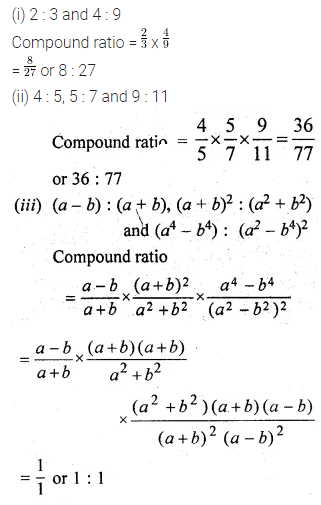Question 3.
Find the duplicate ratio of
(i) 2 : 3
(ii) √5 : 7
(iii) 5a : 6b
Solution: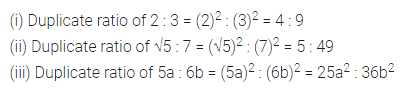Question 4.
Find the triplicate ratio of
(i) 3 : 4
(ii) $$\\ \frac { 1 }{ 2 }$$ : $$\\ \frac { 1 }{ 3 }$$
(iii) 13 : 23
Solution:Question 5.
Find the sub-duplicate ratio of
(i) 9 : 16
(ii) $$\\ \frac { 1 }{ 4 }$$ : $$\\ \frac { 1 }{ 9 }$$,
(iii) 9a2 : 49b2
Solution: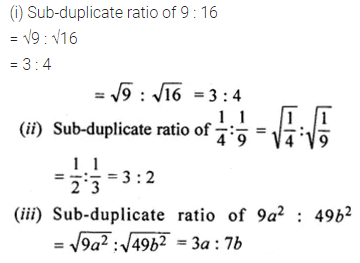Question 6.
Find the sub-triplicate ratio of
(i) 1 : 216
(ii) $$\\ \frac { 1 }{ 8 }$$ : $$\\ \frac { 1 }{ 125 }$$
(iii) 27a3 : 64b3
Solution:Question 7.
Find the reciprocal ratio of
(i) 4 : 7
(ii) 32 : 42
(iii) $$\frac { 1 }{ 9 } :2$$
Solution: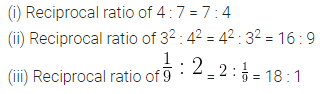Question 8.
Arrange the following ratios in ascending order of magnitude:
2 : 3, 17 : 21, 11 : 14 and 5 : 7
Solution: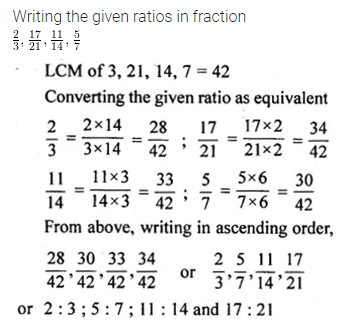Question 9.
(i) If A : B = 2 : 3, B : C = 4 : 5 and C : D = 6 : 7, find A : D
(ii) If x : y = 2 : 3, and y : z = 4 : 7, find x : y : z
Solution: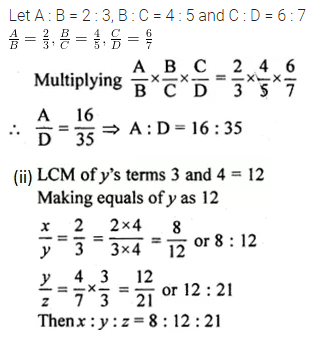Question 10.
(i) If A : B = $$\frac { 1 }{ 4 } :\frac { 1 }{ 5 }$$ and B : C = $$\frac { 1 }{ 7 } :\frac { 1 }{ 6 }$$, find A : B : C.
(ii) If 3A = 4B = 6C, find A : B : C
Solution:Question 11.
(i) If $$\frac { 3x+5y }{ 3x-5y } =\frac { 7 }{ 3 }$$ , Find x : y
(ii) ) If a : b = 3 : 11, find (15a – 3b) : (9a + 5b). a
Solution:Question 12.
(i) If (4x² + xy) : (3xy – y²) = 12 : 5, find (x + 2y) : (2x + y).
(ii) If y (3x – y) : x (4x + y) = 5 : 12. Find (x² + y²) : (x + y)².
Solution: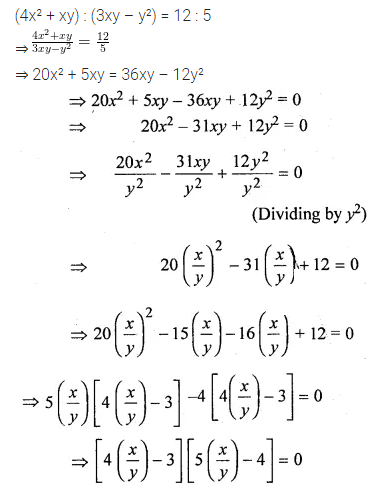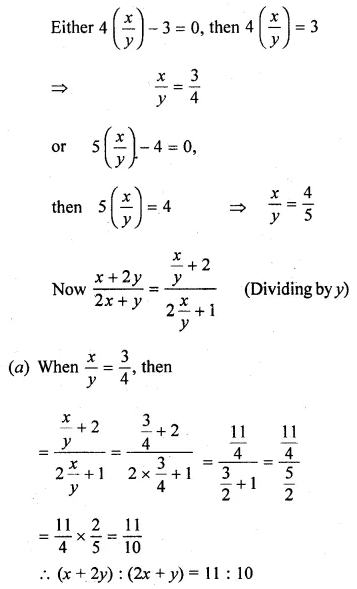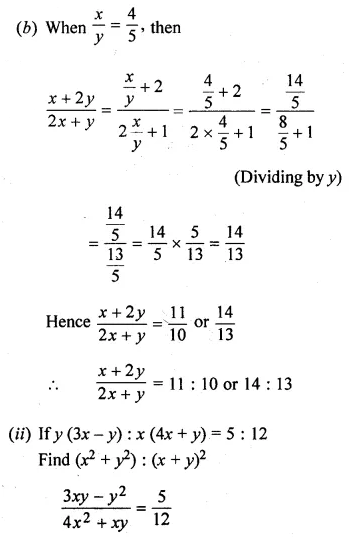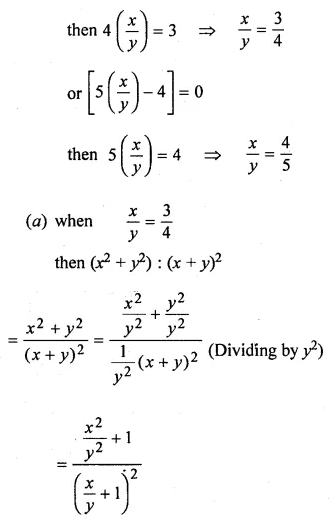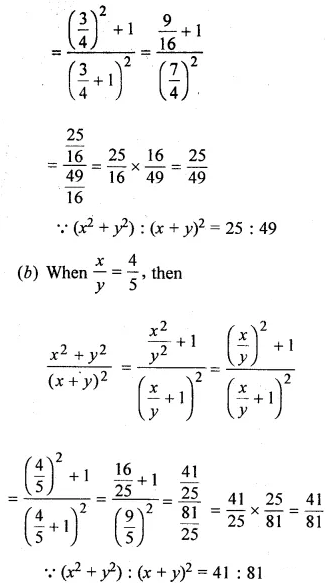Question 13.
(i) If (x – 9) : (3x + 6) is the duplicate ratio of 4 : 9, find the value of x.
(ii) If (3x + 1) : (5x + 3) is the triplicate ratio of 3 : 4, find the value of x.
(iii) If (x + 2y) : (2x – y) is equal to the duplicate ratio of 3 : 2, find x : y.
Solution: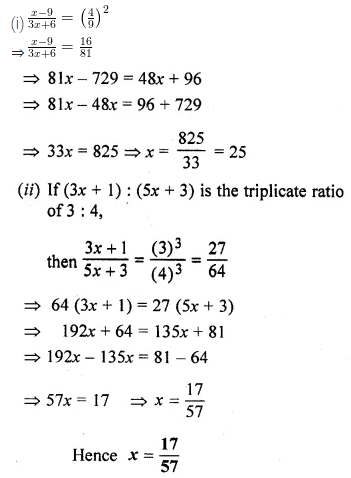Question 14.
(i) Find two numbers in the ratio of 8 : 7 such that when each is decreased by $$12 \frac { 1 }{ 2 }$$, they are in the ratio 11 : 9.
(ii) The income of a man is increased in the ratio of 10 : 11. If the increase in his income is Rs 600 per month, find his new income.
Solution: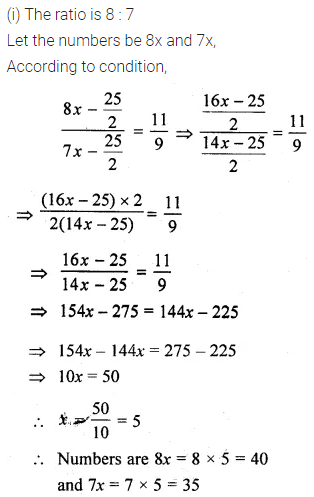Question 15.
(i) A woman reduces her weight in the ratio 7 : 5. What does her weight become if originally it was 91 kg?
(ii) A school collected Rs 2100 for charity. It was decided to divide the money between an orphanage and a blind school in the ratio of 3 : 4. How much money did each receive?
Solution:Question 16.
(i) The sides of a triangle are in the ratio 7 : 5 : 3 and its perimeter is 30 cm. Find the lengths of sides.
(ii) If the angles of a triangle are in the ratio 2 : 3 : 4, find the angles.
Solution: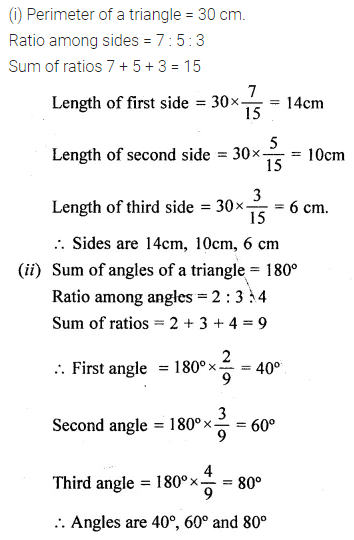Question 17.
Three numbers are in the ratio $$\frac { 1 }{ 2 } :\frac { 1 }{ 3 } :\frac { 1 }{ 4 }$$ If the sum of their squares is 244, find the numbers.
Solution: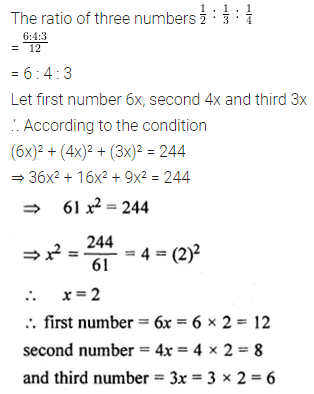Question 18.
(i) A certain sum was divided among A, B and C in the ratio 7 : 5 : 4. If B got Rs 500 more than C, find the total sum divided.
(ii) In a business, A invests Rs 50000 for 6 months, B Rs 60000 for 4 months and C, Rs 80000 for 5 months. If they together earn Rs 18800 find the share of each.
Solution: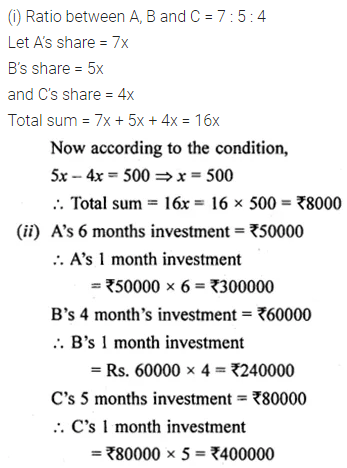Question 19.
(i) In a mixture of 45 liters, the ratio of milk to water is 13 : 2. How much water must be added to this mixture to make the ratio of milk to water as 3 : 1?
(ii) The ratio of the number of boys to the number of girls in a school of 560 pupils is 5 : 3. If 10 new boys are admitted, find how many new girls may be admitted so that the ratio of the number of boys to the number of girls may change to 3 : 2.
Solution: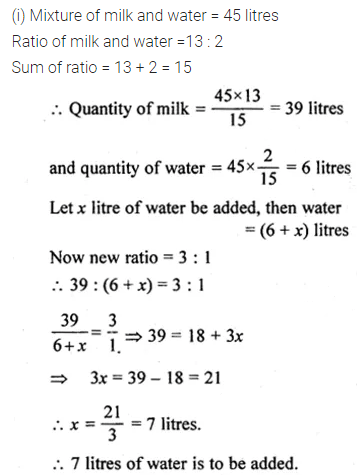Question 20.
(i) The monthly pocket money of Ravi and Sanjeev are in the ratio 5 : 7. Their expenditures are in the ratio 3 : 5. If each saves Rs 80 every month, find their monthly pocket money.
(ii) In class X of a school, the ratio of the number of boys to that of the girls is 4 : 3. If there were 20 more boys and 12 fewer girls, then the ratio would have been 2 : 1, How many students were there in the class?
Solution: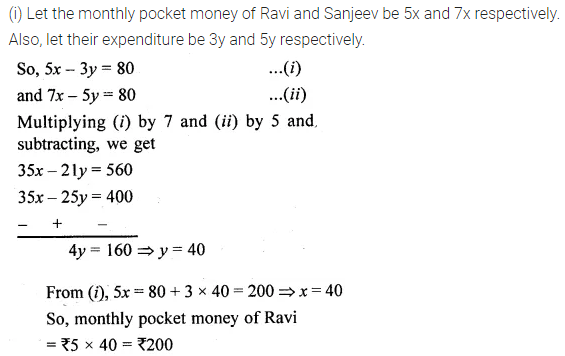Question 21.
In an examination, the ratio of passes to failures was 4 : 1. If 30 less had appeared and 20 less passed, the ratio of passes to failures would have been 5 : 1. How many students appeared for the examination
Solution: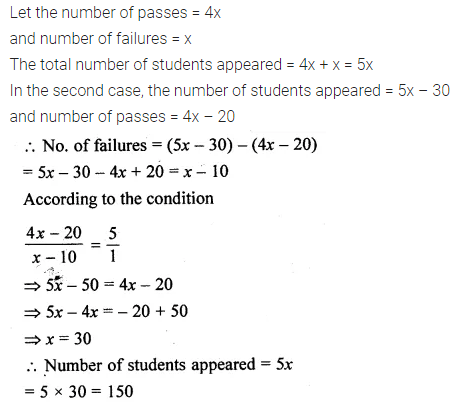ML Aggarwal Class 10 Solutions for ICSE Maths